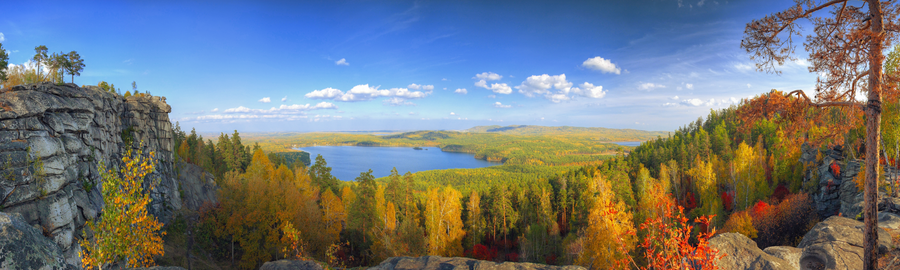# Aspect Ratio `ar`

Resizes and crops the original image to match a specified aspect ratio. This parameter will work only when `fit=crop` is set. Values for `ar` are expressed as `W:H`.• Default: no aspect ratio set. In crop mode, the image is resized to match specified `w` and `h` dimensions exactly, discarding image data as needed. If only one dimension is specified, the other dimension is calculated automatically, preserving the original aspect ratio.

• Aspect ratio set. `ar=9:1&fit=crop`: A 900×270 image cropped using the aspect ratio of 9:1 results in an output image sized 900×100. (Note the same result can be achieved with `w=900&h=100&fit=crop`.) By default, center crop is used; see crop mode for more on directional cropping.`ar=0.85:1&fit=crop:`• Aspect ratio and one dimension set. `w=280&ar=2:1&fit=crop`: Using the aspect ratio of 2:1 and the specified width of 280 results in an output image sized 280×140. The `h` dimension of 140 is automatically calculated.